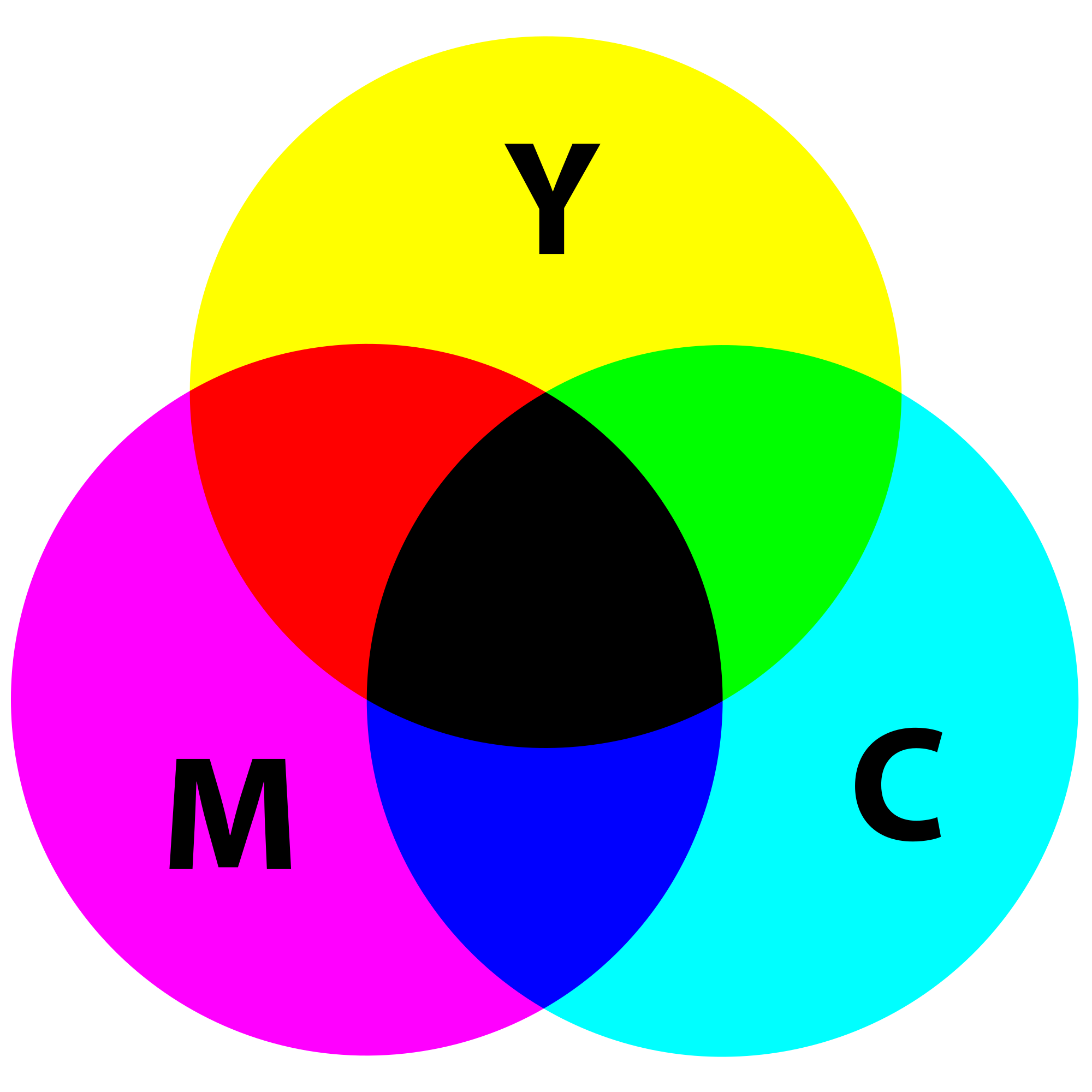# Python | CMY and CMYK Color Models

RGB and HSV, two commonly used color models are discussed in the articles: RGB, HSV. In this article, we introduce the CMY and CMYK color models.

Cyan, magenta and yellow are the secondary colors of light and the primary colors of pigments. This means, if white light is shined on a surface coated with cyan pigment, no red light is reflected from it. Cyan subtracts red light from white light. Unlike the RGB color model, CMY is subtractive, meaning higher values are associated with darker colors rather than lighter ones.Devices that deploy pigments to color paper or other surfaces use the CMY color model, e.g. printers and copiers. The conversion from RGB to CKY is a simple operation, as is illustrated in the Python program below. It is important that all color values be normalized to [0, 1] before converting.

```C = 1 - R
M = 1 - G
Y = 1 - B
```

Below is the code to convert RGB to CMY color model.

 `# Formula to convert RGB to CMY. ` `def` `rgb_to_cmy(r, g, b): ` ` `  `    ``# RGB values are divided by 255  ` `    ``# to bring them between 0 to 1. ` `    ``c ``=` `1` `-` `r ``/` `255` `    ``m ``=` `1` `-` `g ``/` `255` `    ``y ``=` `1` `-` `b ``/` `255` `    ``return` `(c, m, y) ` ` `  `# Sample RGB values. ` `r ``=` `0` `g ``=` `169` `b ``=` `86` ` `  `# Print the result. ` `print``(rgb_to_cmy(r, g, b)) `

Output:

```(1.0, 0.33725490196078434, 0.6627450980392157)
```

According to the color wheel shown above, equal amounts of cyan, magenta, and yellow should produce black. However, in real life, combining these pigments produces a muddy-colored black. To produce pure black, which is quite commonly used while printing, we add a fourth color — black, to the pigment mixture. This is called four-color printing. The addition of black in this model results in it being referred to as the CMYK color model.

My Personal Notes arrow_drop_upCheck out this Author's contributed articles.

If you like GeeksforGeeks and would like to contribute, you can also write an article using contribute.geeksforgeeks.org or mail your article to contribute@geeksforgeeks.org. See your article appearing on the GeeksforGeeks main page and help other Geeks.

Please Improve this article if you find anything incorrect by clicking on the "Improve Article" button below.

Article Tags :

Be the First to upvote.

Please write to us at contribute@geeksforgeeks.org to report any issue with the above content.